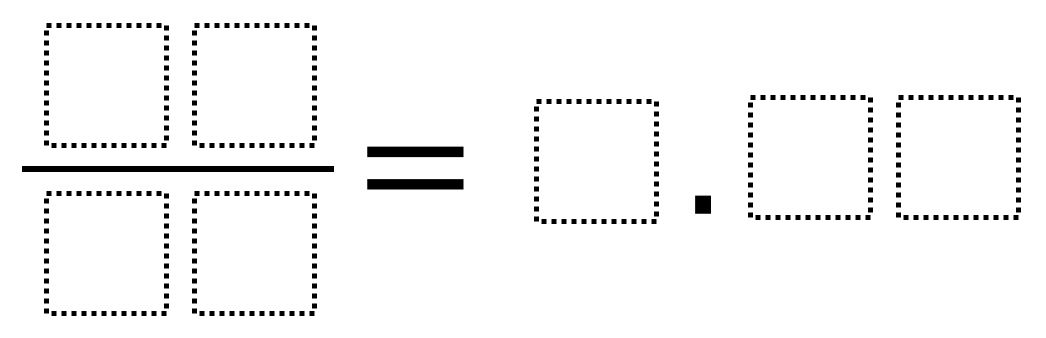Home > Grade 7 > Converting Between Fractions and Decimals

# Converting Between Fractions and Decimals

Directions: Using the numbers 0 through 9, at most one time each, fill in each of the boxes so that the fraction equals the decimal.### Hint

What are easier decimals to find fraction equivalents for?

There are multiple solutions possible including:

17/68 = 0.25

36/48 = 0.75

17/25 = 0.68

72/16 = 4.50

19/25 = 0.76

Source: Robert Kaplinsky

## Converting a Fraction to a Decimal

Directions: Using the digits 1 to 9, at most one time each, place a digit …

1.Also 36/48 = 0.75

•Thank you Sandy for contributing! The solution set has been updated.

•Thanks – my students got a couple more: 17/25=0.68 and 19/25=0.76

•Robert Kaplinsky

Thanks! I added those as well.

2.I think I found another one…72/16 = 4.50

•Robert Kaplinsky

Thanks Daniel. I added it.

•Great Job daniel I got 19/25 = 0.76

3.My class found 4 more unless we are making a mistake!!

18/24= 0.75
73/50= 1.46
48/32= 1.50
80/64= 1.25

•96/25= 3.84

4.Also
39/26 = 1.50
72/48 = 1.50

I really like that this problem has many solutions that can keep kids thinking. Math often has more than one correct answer.

5.76/25=3.04

6.Any statement with using the digit 0 on it?